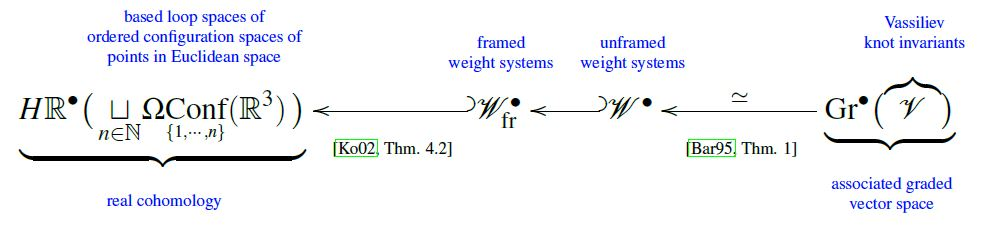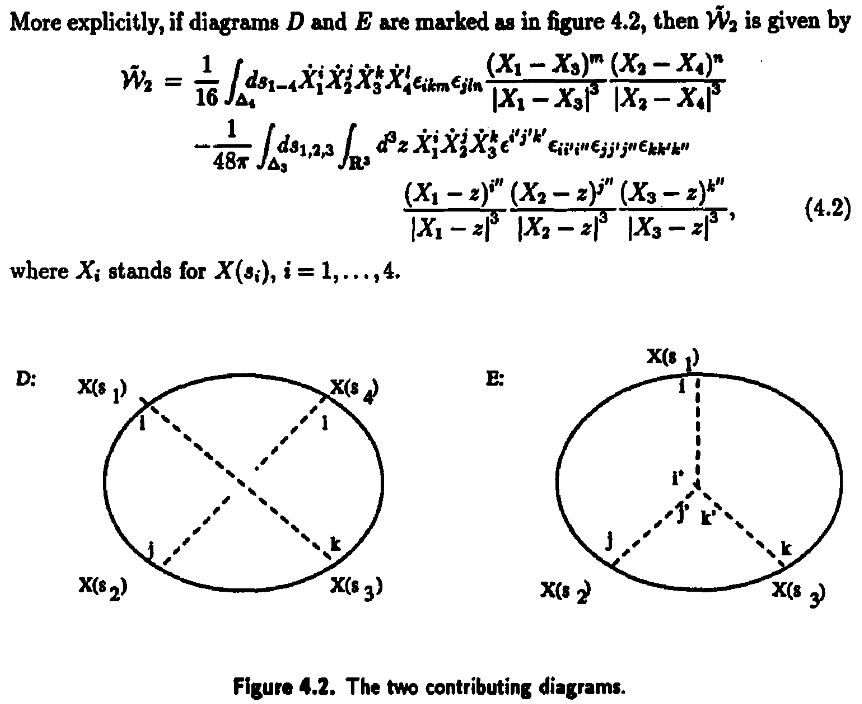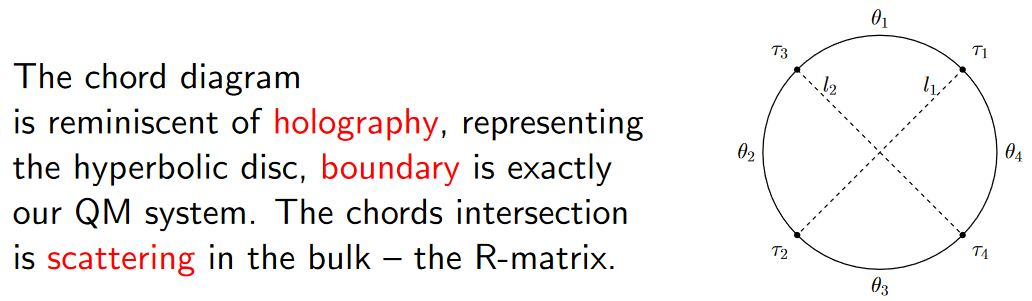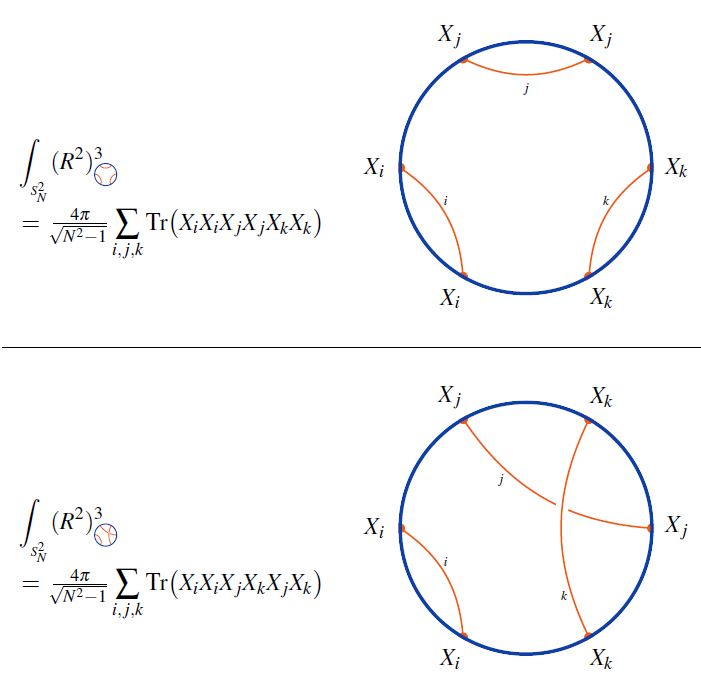# nLab weight system

Contents

### Context

#### Knot theory

knot theory

Examples/classes:

Types

knot invariants

Related concepts:

category: knot theory

# Contents

## Idea

In knot theory a framed weight system is an assignment of numbers to chord diagrams that is invariant under the 4T-relation.

If the assignment is in addition invariant under the 1T-relation, then it is called an unframed weight system, or just weight system, for short.

Similarly, in braid group-theory, a horizontal weight system is an assignment of numbers to horizontal chord diagrams that is invariant under the horizontal 2T relation and horizontal 4T relation.

## Definition

### Round weight systems

###### Definition

(linear span of chord diagrams modulo 4T)

Let $k$ be a field (or just a commutative ring). Write

1. $\mathcal{D}^c$ for the set of chord diagrams,

2. $k\langle \mathcal{D}^c\rangle$ for its $k$-linear span,

3. $\mathcal{A}^c \;\coloneqq\; k\langle \mathcal{D}^c\rangle/4T$ for the quotient vector space by the 4T-relations.

###### Definition

(framed weight system)

A $k$-valued framed weight system is a linear function on the linear span of chord diagrams modulo 4T-relations (Def. )

$w \;\colon\; \mathcal{A}^c \longrightarrow k \,,$

hence the $k$-vector space of framed weight systems is the dual vector space

$\mathcal{W} \;\coloneqq\; (\mathcal{A}^c)^\ast$
###### Remark

Since chord diagrams modulo 4T are Jacobi diagrams modulo STU, framed weight systems equivalently form the dual vector space

(1)\begin{aligned} \overset{ \mathclap{ \color{blue} { {framed} \atop {{weight}\atop{systems}} } } }{ \mathcal{W} } & \;\coloneqq\; \big( \overset { \color{blue} { { {chord} \atop {diagrams} } \atop { {modulo}\,{4T} } } } { \mathcal{A}^c } \big)^\ast \\ & \;\simeq\; \big( \underset{ \color{blue} { { {Jacobi} \atop {diagrams} } \atop { {modulo}\,{STU} } } }{ \mathcal{A}^t } \big)^\ast \end{aligned}

of the quotient vector space $\mathcal{A}^t \coloneqq k\langle \mathcal{D}^t \rangle/STU$ of the linear span of Jacobi diagrams by the STU-relation.

### Horizontal weight systems

###### Definition

(linear span of horizontal chord diagrams modulo 2T/4T)

Let $k$ be a field (or just a commutative ring). Write

1. $\mathcal{D}^{pb}$ for the set of horizontal chord diagrams,

2. $k\langle \mathcal{D}^{pb}\rangle$ for its $k$-linear span,

3. $\mathcal{A}^{pb} \;\coloneqq\; k\langle \mathcal{D}^c\rangle/(2T,4T)$ for the quotient vector space by the 2T relations and horizontal 4T-relations.

(The superscript “${}^{pb}$”in Def. is for pure braids, alluding to the fact that horizontal weight systems are associated graded of Vassiliev braid invaraints.)

###### Definition

(horizontal weight system)

A $k$-valued horizontal weight system is a linear function on the linear span of horizontal chord diagrams modulo 2T&4T-relations (Def. )

$w \;\colon\; \mathcal{A}^{pb} \longrightarrow k \,,$

hence the $k$-vector space of horizontal weight systems is the dual vector space

$\mathcal{W}_{pb} \;\coloneqq\; (\mathcal{A}^{pb})^\ast$

## Constructions of weight systems

### Lie algebra weight systems

A large class of weight systems arises from reading a (horizontal) chord diagram as a string diagram in the evident way, and then labelling it by the structure morphisms of a Lie algebra object equipped with a Lie algebra representation internal to a suitable tensor category. This does yield weight systems because the required relations translate exactly to the structural equations satisfied by Lie modules (Jacobi identity and Lie action property).

The weight systems arising this way are called Lie algebra weight systems. See there for more.

Examples of weight systems which are not Lie algebra weight systems are rare. Originally it was conjectured that none exist (Bar-Natan 95, Conjecture 1, Bar-Natan & Stoimenow 97, Conjecture 2.4).

Eventually, a (counter-)example of a weight system which at least does not arise from any finite-dimensional super Lie algebra was given in Vogel 11.

### Stringy weight systems

stringy weight systems

### Rozansky-Witten weight systems

Rozansky-Witten weight systems

## Properties

### As the associated graded space of Vassiliev invariants

###### Proposition

(weight systems are associated graded of Vassiliev invariants)

For ground field $k = \mathbb{R}, \mathbb{C}$ the real numbers or complex numbers, there is for each natural number $n \in \mathbb{N}$ a canonical linear isomorphism

$\mathcal{V}_n/\mathcal{V}_{n-1} \underoverset{\simeq}{\phantom{AAAA}}{\longrightarrow} \big( \mathcal{A}_n^u \big)^\ast$

from

1. the quotient vector space of order-$n$ Vassiliev invariants of knots by those of order $n-1$

2. to the space of unframed weight systems of order $n$.

In other words, in characteristic zero, the graded vector space of unframed weight systems is the associated graded vector space of the filtered vector space of Vassiliev invariants.

(Bar-Natan 95, Theorem 1, following Kontsevich 93)

### As cohomology of loop spaces of configuration spaces

#### For horizontal chord diagrams

###### Proposition

(integral horizontal weight systems are integral cohomology of based loop space of ordered configuration space of points in Euclidean space)

For ground ring $R = \mathbb{Z}$ the integers, there is, for each natural number $n$, a canonical isomorphism of graded abelian groups between

1. the integral weight systems

$\mathcal{W}_{pb} \;\coloneqq\; Hom_{\mathbb{Z} Mod} \big( \underset{ \mathcal{A}^{pb} }{ \underbrace{ \mathbb{Z} \langle \mathcal{D}^{pb} \rangle /(2T,4T) } } , \mathbb{Z} \big)$

on horizontal chord diagrams of $n$ strands (elements of the set $\mathcal{D}^{pb}$)

$H \mathbb{Z}^\bullet \big( \underset{n \in \mathbb{N}}{\sqcup} \Omega \underset{ {}^{\{1,\cdots,n\}} }{Conf} (\mathbb{R}^3) \big) \;\simeq\; (\mathcal{W}_{pb})^\bullet \;\simeq\; Gr^\bullet( \mathcal{V}_{pb}) \,.$

(the second equivalence on the right is the fact that weight systems are associated graded of Vassiliev invariants).

This is stated as Kohno 02, Theorem 4.1

#### For round chord diagrams

###### Proposition

(weight systems are inside real cohomology of based loop space of ordered configuration space of points in Euclidean space)

For ground field $k = \mathbb{R}$ the real numbers, there is a canonical injection of the real vector space $\mathcal{W}$ of framed weight systems (here) into the real cohomology of the based loop spaces of the ordered configuration spaces of points in 3-dimensional Euclidean space:

$\mathcal{W} \;\overset{\;\;\;\;}{\hookrightarrow}\; H\mathbb{R}^\bullet \Big( \underset{n \in \mathbb{N}}{\sqcup} \Omega \underset{{}^{\{1,\cdots,n\}}}{Conf} \big( \mathbb{R}^3 \big) \Big)$

This is stated as Kohno 02, Theorem 4.2

Combining the above two propositions

we get this situation:### As cohomology of the knot graph complex

cohomology of knot graph complex is weight systems on chord diagrams

### General

Original articles:

Textbook accounts:

### Lie algebra weight systems

Discussion of Lie algebra weight systems

From the construction given in Bar-Natan 95, Section 2.4 the interpretation of Lie algebra weight systems in terms of string diagrams for Lie algebra objects in tensor categories is evident, but standard textbooks in knot theory/combinatorics do not pick this up:

The interpretation of Lie algebra weight systems as string diagram-calculus and generalization to Lie algebra objects (motivated by generalization at least to super Lie algebras) is made more explicit in

and fully explicit in

• Vladimir Hinich, Arkady Vaintrob, Cyclic operads and algebra of chord diagrams, Sel. math., New ser. (2002) 8: 237 (arXiv:math/0005197)

• E. Kulakova, S. Lando, T. Mukhutdinova, G. Rybnikov, On a weight system conjecturally related to $\mathfrak{sl}_2$, European Journal of Combinatorics Volume 41, October 2014, Pages 266-277 (arXiv:1307.4933)

• Alexander Schrijver, On Lie algebra weight systems for 3-graphs (arXiv:1412.6923)

### As the associated graded space of Vassiliev invariants

On spaces of weight systems as the associated graded spaces of Vassiliev invariants:

### As cohomology of loop spaces of configuration spaces

On weight systems as the real cohomology of based loop spaces of ordered configuration spaces of points:

### Chord diagrams and weight systems in Physics

The following is a list of references that involve (weight systems on) chord diagrams/Jacobi diagrams in physics:

For a unifying perspective and further pointers see:

#### In Chern-Simons theory

Since weight systems are the associated graded of Vassiliev invariants, and since Vassiliev invariants are knot invariants arising as certain correlators/Feynman amplitudes of Chern-Simons theory in the presence of Wilson lines, there is a close relation between weight systems and quantum Chern-Simons theory.

Historically this is the original application of chord diagrams/Jacobi diagrams and their weight systems, see also at graph complex and Kontsevich integral.Reviewed in:

Applied to Gopakumar-Vafa duality:

• Dave Auckly, Sergiy Koshkin, Introduction to the Gopakumar-Vafa Large $N$ Duality, Geom. Topol. Monogr. 8 (2006) 195-456 (arXiv:0701568)

#### For single trace operators in AdS/CFT duality

Interpretation of Lie algebra weight systems on chord diagrams as certain single trace operators, in particular in application to black hole thermodynamics

#### In $AdS_2/CFT_1$, JT-gravity/SYK-model

Discussion of (Lie algebra-)weight systems on chord diagrams as SYK model single trace operators:

• Antonio M. García-García, Yiyang Jia, Jacobus J. M. Verbaarschot, Exact moments of the Sachdev-Ye-Kitaev model up to order $1/N^2$, JHEP 04 (2018) 146 (arXiv:1801.02696)

• Yiyang Jia, Jacobus J. M. Verbaarschot, Section 4 of: Large $N$ expansion of the moments and free energy of Sachdev-Ye-Kitaev model, and the enumeration of intersection graphs, JHEP 11 (2018) 031 (arXiv:1806.03271)

• Micha Berkooz, Prithvi Narayan, Joan Simón, Chord diagrams, exact correlators in spin glasses and black hole bulk reconstruction, JHEP 08 (2018) 192 (arxiv:1806.04380)

following:

• László Erdős, Dominik Schröder, Phase Transition in the Density of States of Quantum Spin Glasses, D. Math Phys Anal Geom (2014) 17: 9164 (arXiv:1407.1552)

which in turn follows

• Philippe Flajolet, Marc Noy, Analytic Combinatorics of Chord Diagrams, pages 191–201 in Daniel Krob, Alexander A. Mikhalev,and Alexander V. Mikhalev, (eds.), Formal Power Series and Algebraic Combinatorics, Springer 2000 (doi:10.1007/978-3-662-04166-6_17)With emphasis on the holographic content:

• Micha Berkooz, Mikhail Isachenkov, Vladimir Narovlansky, Genis Torrents, Section 5 of: Towards a full solution of the large $N$ double-scaled SYK model, JHEP 03 (2019) 079 (arxiv:1811.02584)

• Vladimir Narovlansky, Slide 23 (of 28) of: Towards a Solution of Large $N$ Double-Scaled SYK, 2019 (pdf)

and specifically in relation, under AdS2/CFT1, to Jackiw-Teitelboim gravity:

#### In D$p$/D$(p+2)$-brane intersectionsgraphics from Sati-Schreiber 19c

#### As codes for holographic entanglement entropy

Chord diagrams encoding Majorana dimer codes and other quantum error correcting codes via tensor networks exhibiting holographic entanglement entropy: